# Fourier Series

1 Introduction 2 Periodic Functions 3 Even And Odd Functions 4 Half Range Series 5 ParsevalŌĆÖs Theorem 6 Change Of Interval 7 Harmonic Analysis 8 Complex Form Of Fourier Series 9 Summary

FOURIER SERIES

1 INTRODUCTION

2 PERIODIC FUNCTIONS

3 EVEN AND ODD FUNCTIONS

4 HALF RANGE SERIES

5 PARSEVALŌĆÖS THEOREM

6 CHANGE OF INTERVAL

7 HARMONIC ANALYSIS

8 COMPLEX FORM OF FOURIER SERIES

9 SUMMARY

1 INTRODUCTION

The concept of Fourier series was first introduced by Jacques Fourier (1768ŌĆō 1830), French Physicist and Mathematician. These series became a most important tool in Mathematical physics and had deep influence on the further development of mathematics it self.Fourier series are series of cosines and sines and arise in representing general periodic functions that occurs in many Science and Engineering problems. Since the periodic functions are often complicated, it is necessary to express these in terms of the simple periodic functions of sine and cosine. They play an important role in solving ordinary and partial differential equations.

2 PERIODIC FUNCTIONS

A function f (x) is called periodic if it is defined for all real ŌĆ×xŌĆ¤ some positive number ŌĆ×pŌĆ¤ such that

f (x + p ) = f (x) for all x.

This         number   ŌĆ×pŌĆ¤   is   called   a   period   of   f(x

If a periodic function f (x) has a smallest period p (>0), this is often called the fundamental period of f(x). For example, the functions cosx and sinx have fundamental period 2p.

DIRICHLET CONDITIONS

Any function f(x), defined in the interval c ┬Ż┬Żc + 2p, can be developed as a Fourier series of the form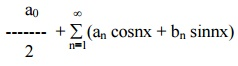provided the following conditions are satisfied.

f (x) is periodic, singleŌĆōvalued and finite in [ c, c + 2 p].

f (x) has a finite number of discontinuities in [ c, c + 2p].

f (x) has at the most a finite number of maxima and minima in [ c,c+ 2p].

These conditions are known as Dirichlet conditions. When these conditions are satisfied, the Fourier series converges to f(x) at every point of continuity. At a point of discontinuity x = c, the sum of the series is given by

f(x) = (1/2) [ f (c-0) + f (c+0)] ,

where f (c-0) is the limit on the left and f (c+0) is the limit on the right.

EULERŌĆÖS  FORMULAE

The Fourier series for the function f(x) in the interval c < x < c + 2pis given byThese values of a0, an, bn are   known   as   EulerŌĆ¤s 0 , formula en,b The coefficients a0, an, bn  are  are also termed as Fourier coefficients.

Example 1

Expand f(x) = x as Fourier Series (Fs)  in the interval [ -ŽĆ,   ŽĆ]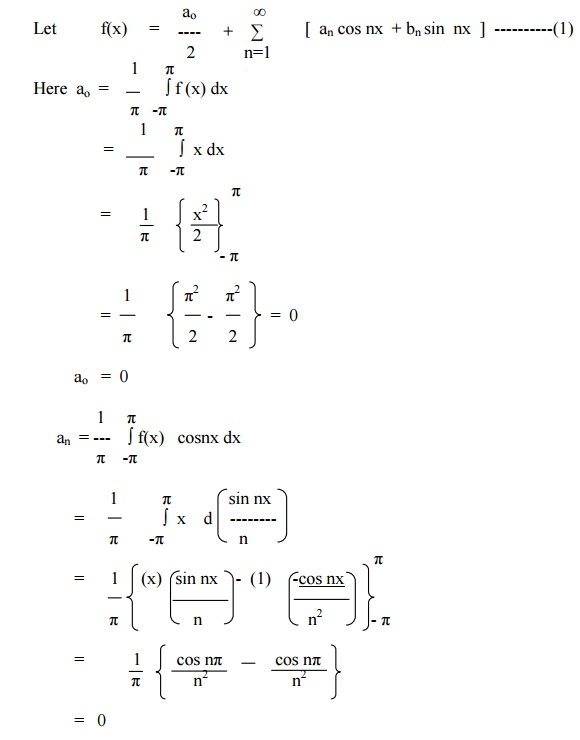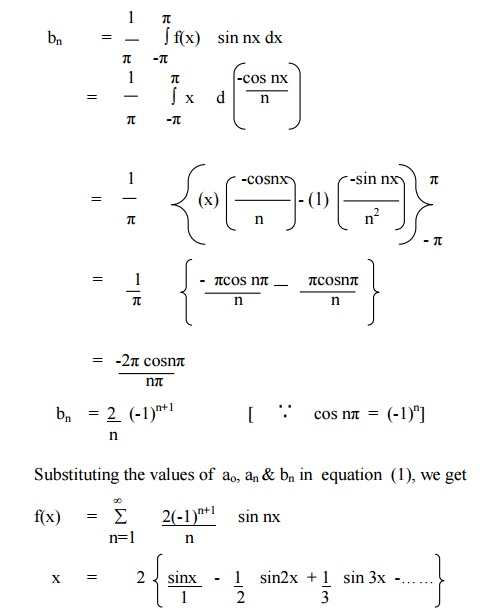Example 2

Expand   f(x) = xin the interval ( -p┬Ż┬Żp) and hence deduce thatExample 3

Obtain the Fourier Series of periodicity   2ŽĆin [for-ŽĆ, ŽĆ]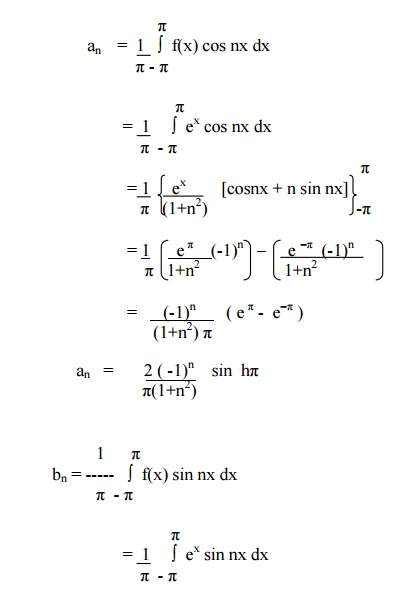Example 4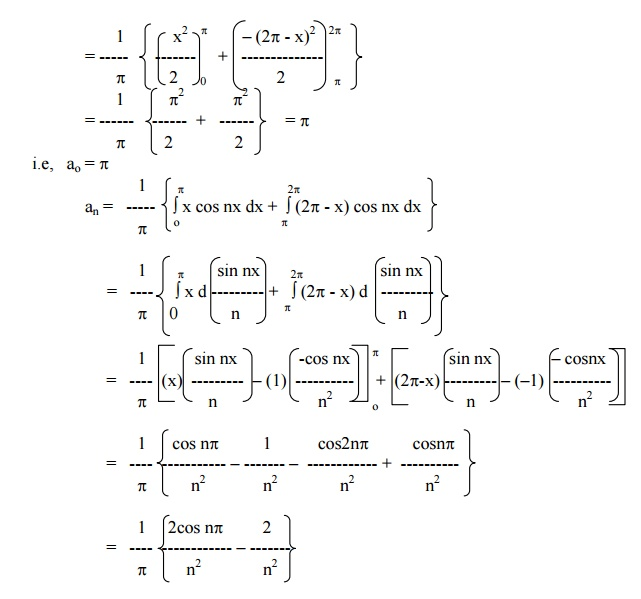Example 5

Find the Fourier series for f (x) = (x + x2) in (-p< x < p) of percodicity 2and hence deduce thatHere x = -pand x = pare the end points of the range. \ The value of FS at x = pis the average of the values of f(x) at x = pand x = -p.Exercises:

Determine the Fourier expressions of the following functions in the given interval

1.f(x) = (p- x)2, 0 < x < 2p

2.f(x) = 0 in -p< x < 0

pin 0 < x < p

3.f(x) = x ŌĆōx2 in [-p,p

4.f(x) = x(2p-x) in (0,2p

5.f(x) = sinh ax in [-pp

6.f(x) = cosh ax in [-pp

7.f(x) = 1 in 0 < x < p

= 2 in p< x < 2p

8.f(x) = -p/4 when -p< x < 0

=  p/4 when 0 < x < p

9.f(x) = cosax, in -p< x < p,   whereaŌĆ¤isŌĆ× not   an   integer

10.Obtain a fourier series to represent e-ax from x = -pto x = p. Hence derive the series for p/ sinhp

3 Even and Odd functions

A function f(x) is said to be even if f (-x) = f (x). For example x2, cosx, x sinx, secx are even functions. A function f (x) is said to be odd if f (-x) = - f (x). For example, x3, sin x, x cos x,. are odd functions.

(1) The EulerŌĆ¤s formula for even function is a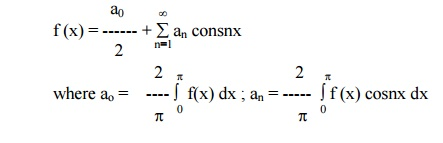(2) The   EulerŌĆ¤s   formula   for   odd   function   isExample 6

Find the Fourier Series for f (x) = x in ( -pp)

Here, f(x) = x        is an odd function.Example 7

Expand f (x) = |x| in (-pp) as FS and hence deduce thatExample 8Then find the FS for f(x) and hence show thatHere f (-x) in (-p,0) = f (x) in (0,p)

f  (-x) in (0,p) = f (x) in (-p,0)

f(x) is a even functionExample 9

Obtain the FS expansion of f(x) = x sinx in (-p< x<p) and hence deduce thatHere f (x) = xsinx  is an even function.Exercises:

Determine Fourier expressions of the following functions in the given interval:

i. f(x) = p/2 + x, -p< x < 0

p/2 - x, 0 < x < p

ii. f(x) = -x+1 for + -p< x < 0

x+1 for 0 =< x =< p4 HALF RANGE SERIES

It is often necessary to obtain a Fourier expansion of a function for the range (0, p) which is half the period of the Fourier series, the Fourier expansion of such a function consists a cosine or sine terms only.

(i) Half Range Cosine Series

The Fourier cosine series for f(x) in the interval (0,p) is given by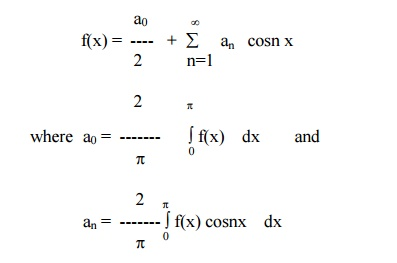(ii)    Half Range Sine Series

The Fourier sine series for f(x) in the interval (0,p) is given byExample 10

If  c is the constant in ( 0 < x < p) then show that

c = (4c / p)  { sinx + (sin3x /3) + sin5x / 5) + ... ... ... }

Given   f(x) = c in (0,p).Example 11

Find the Fourier Half Range Sine Series and Cosine Series for f(x) = x in the interval (0,p).

Sine Series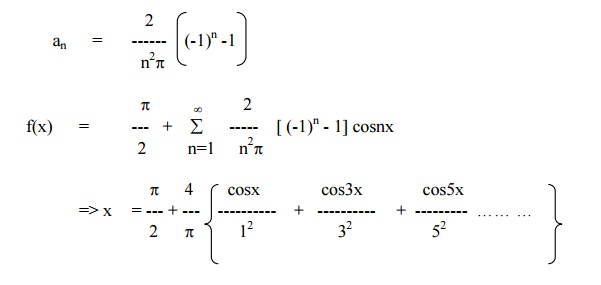Example 12

Find the sine and cosine half-range series for the function function . f(x) = x , 0 < x ┬ŻŽĆ/2

= ŽĆ-x,  ┬Żx< pŽĆ/2

Sine series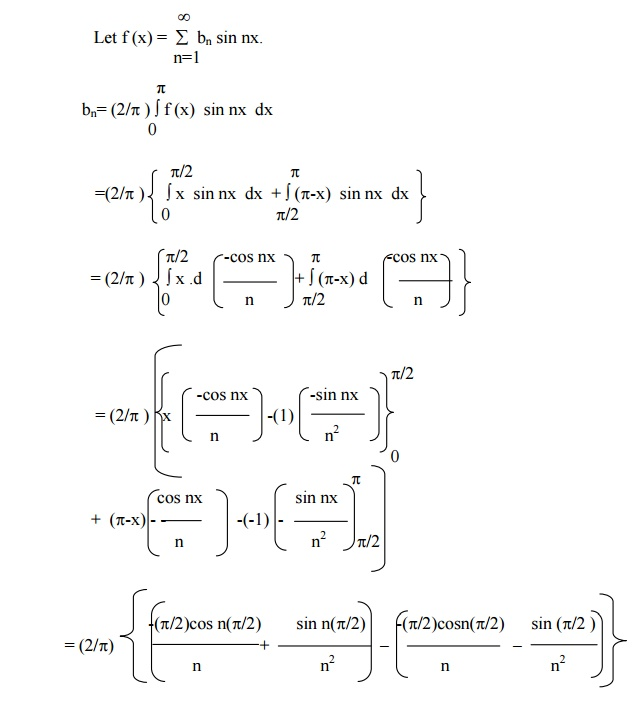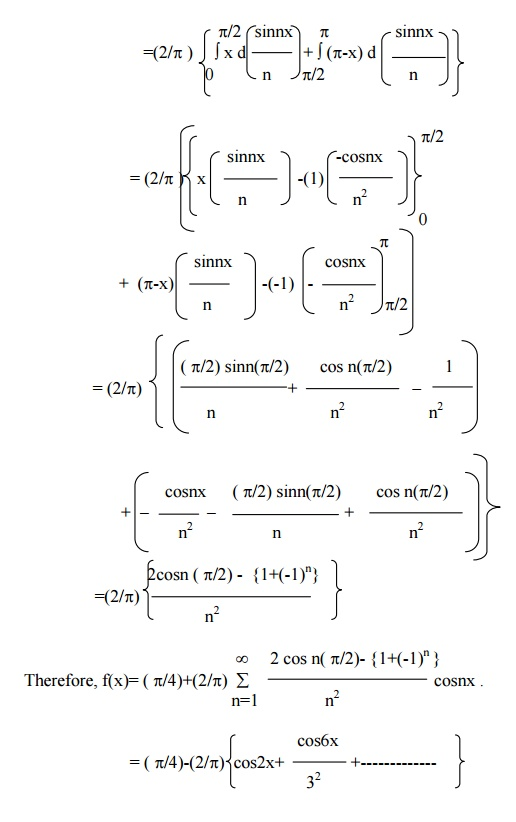Exercises

1.Obtain cosine and sine series for f(x) = x in the interval 0< x < p. Hence show that 1/122.Find the half range cosine and sine series for f(x) = x2 in the range 0 < x < p

3.Obtain the half-range cosine series for the function f(x) = xsinx in (0,p)..

4.Obtain cosine and sine series for f(x) = x (p-x) in 0< x < p 5.Find the half-range cosine series for the function

6.f(x) = (px) / 4 , 0<x< (p/2)

= (p/4)(p-x), p/2 < x < p.

7.Find half range sine series and cosine series for

f(x) = x in  0<x< (p/2)

= 0 in  p/2 < x < p.

8.Find half range sine series and cosine series for the function f(x) == p- x in the interval 0 < x < p.

9.Find the half range sine series of f(x) = x cosx in (0,p)

10.Obtain cosine series for

f(x) = cos x ,   0<x< (p/2)

=   0,  p/2 < x < p

5 ParsevalŌĆÖs Theorem

Root Mean square value of the function f(x) over an interval (a, b) is defined asThe use  of r.m.s value of a periodic  function is frequently made in the theory of mechanical vibrations and in electric circuit theory. The r.m.s value  is known as the effective value of the  function.

ParsevalŌĆÖs   Theorem

If f(x) defined in the interval (c, c+2ŽĆ ), then the ParsevalŌĆ¤s Identity is given byExample 13

Obtain the Fourier series for f(x)CHANGE OF INTERVAL

In most of the Engineering applications, we require an expansion of a given function over an interval 2l other than 2p.

Suppose f(x) is a function defined in the interval c< x < c+2l. The Fourier expansion for f(x) in the interval c<x<c+2l is given byEven and Odd Function

If f(x) is an even function and is defined in the interval ( c, c+2 l ), thenHalf Range Series

Sine SeriesCosine seriesExample 14

Find the Fourier series expansion for the function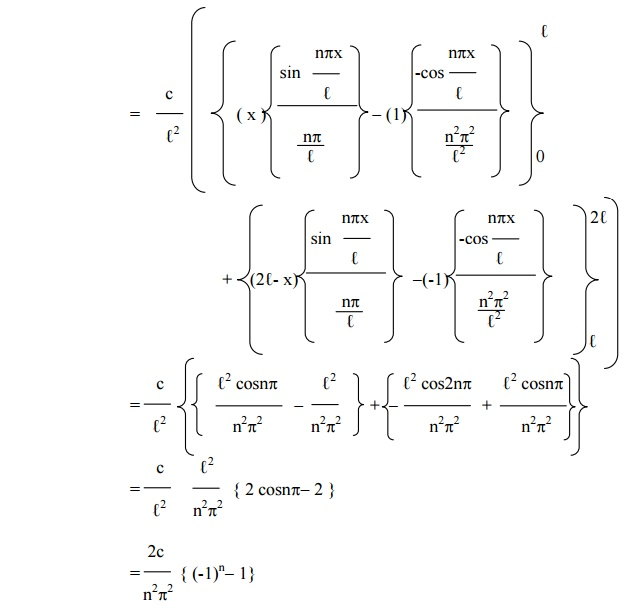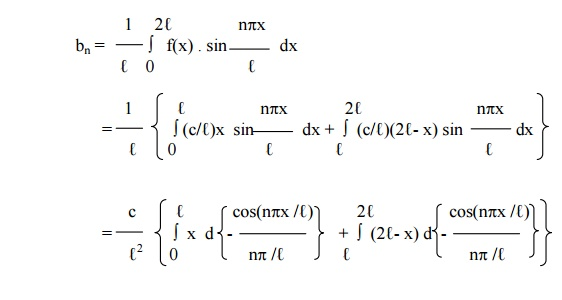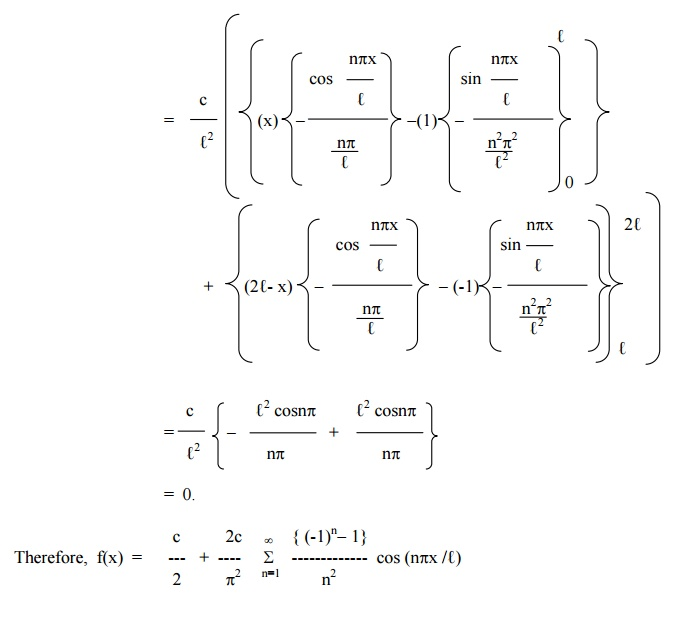Example 15

Find the Fourier series of periodicity 3 for f(x) = 2x ŌĆōx2 , in 0 <<3.

Here 2Ōäō = 3.

\ Ōäō = 3 / 2.Exercises

1.Obtain the Fourier series for f(x) = px in 0 < x < 2. 2.Find the Fourier series to represent x2 in the interval (-l, l ). 3.Find a Fourier series in (-2, 2), if

f(x) = 0, -2 < x < 0

= 1, 0 < x < 2.

4.Obtain the Fourier series for

f(x) =          1-x in 0 < x < l

= 0 in  l      < x < 2 l.     Hence deduce that

1- (1/3 ) +(1/5) ŌĆō(1/7) p/4+&  ŌĆ”   =

2                2        ) + (1/5        2                 2

(1/1 ) + (1/3 )   +  pŌĆ”/8) =   (

5.If f(x) = px,                          0 < x < 1

p (2-x), 1 < x < 2,

Show that in the interval (0,2),6.Obtain the Fourier series for

f(x) = x in 0 < x < 1

= 0 in 1 < x < 2

7.Obtain the Fourier series for

f(x) =          (cx /l ) in 0 < x < l

=       (c/l  ) (2 l  - x ) in l          < x < 2 l .

8.Obtain the Fourier series for

f(x) = (l + x ), - l < x < 0. = (l - x ), 0 < x < l.10.Express f(x) = x  as a half ŌĆōrange sine series in 0 < x < 2

11.Obtain the half-range  sine series for ex in 0 < x < 1.

12.Find the half ŌĆōrange cosine series for the function f(x) = (x-2)2 in the interval 0 < x < 2.

7 Harmonic Analysis

The process of finding the Fourier series for a function given by numerical values is known as harmonic analysis.ie, f(x) = (a0/2) + (a1 cosx + b1 sinx) + (a2 cos2x + b2 sin2x) + (a3cos3x + b3sin3x)+-------------ŌĆ”(1)In (1), the term (a1cosx + b1 sinx) is called the fundamental or first harmonic, the term (a2cos2x + b2sin2x) is called the second harmonic and so on.

Example 16

Compute the first three harmonics of the Fourier series of f(x) given by the following table.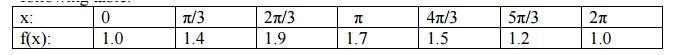We    exclude   the   last   point   x   =   2ŽĆ.

Let  f(x) = (a0/2) + (a1 cosx + b1 sinx) + (a2 cos2x + b2 sin2x) + ŌĆ”ŌĆ”ŌĆ”ŌĆ”

To evaluate the coefficients, we form the following table.\ f(x) = 1.45 ŌĆō 0.37cosx + 0.17 sinx ŌĆō 0.1cos2x ŌĆō 0.06 sin2x + 0.033 cos3x+ŌĆ”

Example 17

Obtain the first three coefficients in the Fourier cosine series for y, where y is given in the following table:\ Fourier cosine series in the interval (0, 2ŽĆ) is y = (a0 /2) + a1cosq+ a2cos2q+ a3cos3q+ ŌĆ”..

To evaluate the coefficients, we form the following table.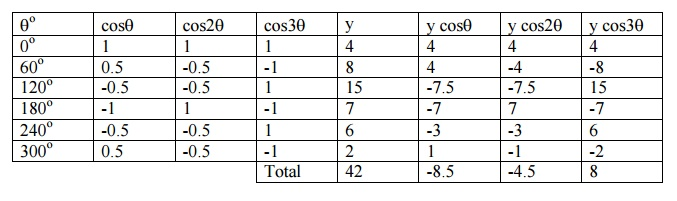Now,         a0 = 2 (42/6) = 14

a1 = 2 ( -8.5/6) = - 2.8

a2 = 2        (-4.5/6) =

a3 = 2 (8/6) = 2.7

y = 7 ŌĆō2.8 cosq- 1.5 cos2q+ 2.7 cos3q+   ŌĆ”..

Example 18

The values of x and the corresponding values of f(x) over a period T are given below. Show that  f(x) = 0.75 + 0.37 cosq+ 1.004 sinq,where q= (2ŽĆx   )/TWe omit the last value since f(x) at x = 0 is known.

Here q= 2ŽĆx / T

When x varies from 0 to T, qvaries from   0   to   2ŽĆ   with2ŽĆ/6.   an   incre

Let  f(x) = F(q) = (a0/2) + a1 cosq+ b1 sinq.

To evaluate the coefficients, we form the following table.Now, a0  = 2 ( Ōłæ   f(x)/6)=1.5

a1 = 2 (1.12 /6) = 0.37

a2 = 2 (3.013/6) =  1.004

Therefore, f(x) = 0.75 + 0.37 cosq+ 1.004 sinq

Exercises

1.The following table gives the variations of periodic current over a period.

t (seconds)   :        0        T/6    T/3    T/2    2T/3  5T/6  T

A (amplitude): 1.98       1.30   1.05   1.30   -0.88 -0.25 1.98

Show that there is a direct current part of 0.75 amp in the variable current and obtain the

amplitude of the first harmonic.

2.The turning moment  T is given for a series of values of the crank angle q┬░=75┬░

q┬░      :         0        30      60      90      120    150    180

T┬░      :         0        5224  8097  7850  5499  2626  0

Obtain the first four terms in a series of sines to represent T and calculate

T for q= 75┬░

3. Obtain the constant term and the co-efficient of the first sine and cosine terms in the

Fourier   expansionfollowingof tableŌĆ×yŌĆ¤.   as   given   in   th

X       :         0        1        2        3        4        5

Y       :         9        18      24      28      26      20

4. Find the first three harmonics of Fourier series of y = f(x) from the following data.

X : 0 ┬░ 30┬░ 60┬░ 90┬░ 120┬░ 150┬░ 180┬░ 210┬░ 240┬░ 270┬░ 300┬░ 330┬░

Y : 298  356373  337     254    15580         516093       147    221

2.8 Complex Form of Fourier Series

The series for f(x) defined in the        interval (c, c+2ŽĆ)and satisfying

8 Complex Form of Fourier Series

The series for f(x) defined in the        interval (c, c+2ŽĆ)and satisfying

DirichletŌĆ¤s conditions can be given in the form ofIn the interval (c, c+2Ōäō), the complex  form of Fourier series is given byExample 19

Find the complex form of the Fourier series  f(x)  = e ŌĆōx  in  -1   Ōēż   x         Ōēż   1.Example 20

Find the complex  form of the Fourier series f(x)  = ex in  - ŽĆ   <   x<   ŽĆ.Exercises

Find the complex form of the Fourier series of the following functions.

1.f(x) = eax, -l < x < l.

2.f(x) = cosax, -p< x < p.

3.f(x) = sinx, 0 < x < p.

4.f(x) = e-x, -1 < x < 1.

5.f(x) = sinax, a is not an integer in (-pp).

9 SUMMARY(FOURIER SERIES)

A Fourier series of a periodic function consists of a sum of sine and cosine terms. Sines and cosines are the most fundamental periodic functions.The Fourier series is named after the French Mathematician and Physicist Jacques Fourier (1768 ŌĆō1830). Fourier series has its application in problems pertaining to Heat conduction, acoustics, etc. The subject matter may be divided into the following sub topics.FORMULA FOR FOURIER SERIES

Consider a real-valued function f(x) which obeys the following conditions called

DirichletŌĆ¤s conditions   :

1.     f(x) is defined in an interval (a,a+2l), and f(x+2l) = f(x) so that f(x) is a periodic function of period 2l

2.     f(x) is continuous or has only a finite number of discontinuities in the interval (a,a+2l).

3.     f(x) has no or only a finite number of maxima or minima in the interval (a,a+2l).

Also, letis called the Fourier series of f(x) in the interval (a,a+2l). Also, the real numbers a0, a1, a2, ŌĆ”.an, and b1, b2 , ŌĆ”.bare called the Fourier coefficients of f(x). The formulae (1), (2) and   (3)   are   called   EulerŌĆ¤s   formulae.

It can be proved that the sum of the series (4) is f(x) if f(x) is continuous at x. Thus we have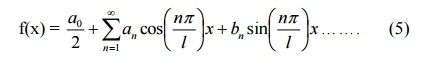Suppose f(x) is discontinuous at x, then the sum of the series (4) wouldwhere f(x+) and f(x-) are the values of f(x) immediately to the right and to the left of f(x) respectively.

Particular Cases Case (i)

Suppose a=0. Then f(x) is defined over the interval (0,2l). Formulae (1), (2), (3) reduce toThen the right-hand side of (5) is the Fourier expansion of f(x) over the interval (0,2l).

If we set l=p, then f(x) is defined over the interval (0,2p). Formulae (6) reduce to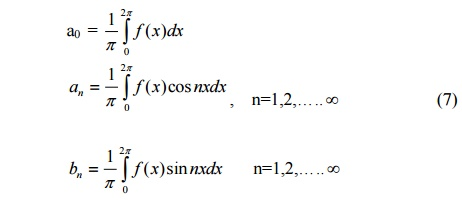Also, in this case, (5) becomesCase (ii)

Suppose a=-l. Then f(x) is defined over the interval (-l , l). Formulae (1), (2) (3) reduce toThen the right-hand side of (5) is the Fourier expansion of f(x) over the interval (-l , l).

If we set l = p, then f(x) is defined over the interval (-pp).  Formulae (9) reduce toSome useful results :

1.     The   following   rule   called   BernoulliŌĆ¤seful in evaluating the Fourier coefficients.2.       The following integrals are also useful :3.                                         If   ŌĆ×nŌĆ¤   is   integer,   then

sin np= 0 ,       cosnp= (-1)n ,        sin2np= 0,  cos2np=1

ASSIGNMENT

1. The displacement y of a part of a mechanism is tabulated with corresponding angular movement x0 of the crank. Express y as a Fourier series upto the third harmonic.

x0      0        30      60      90      120    150    180    210    240    270    300    330

Y       1.80   1.10   0.30   0.16   1.50   1.30   2.16   1.25   1.30   1.52   1.76   2.00

2. Obtain the Fourier series of y upto the second harmonic using the following table :

x0      45      90      135    180    225    270    315    360

y        4.0     3.8     2.4     2.0     -1.5   0        2.8     3.4

3. Obtain the constant term and the coefficients of the first sine and cosine terms in the Fourier expansion of y as given in the following table :

x        0        1        2        3        4        5

y        9        18      24      28      26      20

4. Find the Fourier series of  y upto the second harmonic from the following table :

x        0        2        4        6        8        10      12

Y       9.0     18.2   24.4   27.8   27.5   22.0   9.0

5. Obtain the first 3 coefficients in the Fourier cosine series for y, where y is given below

x        0        1        2        3        4        5

y        4        8        15      7        6        2

Study Material, Lecturing Notes, Assignment, Reference, Wiki description explanation, brief detail
Mathematics (maths) : Fourier Series : Fourier Series |